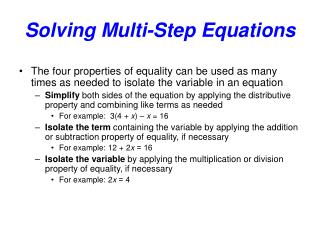DownloadDownload PresentationSolving Multi-Step Equations

# Solving Multi-Step Equations

Download Presentation## Solving Multi-Step Equations

- - - - - - - - - - - - - - - - - - - - - - - - - - - E N D - - - - - - - - - - - - - - - - - - - - - - - - - - -
##### Presentation Transcript

1. Solving Multi-Step Equations • The four properties of equality can be used as many times as needed to isolate the variable in an equation • Simplify both sides of the equation by applying the distributive property and combining like terms as needed • For example: 3(4 + x) – x = 16 → 12 + 3x – x = 16 → 12 + 2x = 16 • Isolate theterm containing the variable by applying the addition or subtraction property of equality, if necessary • For example: 12 + 2x = 16 → 12 + 2x – 12 = 16 – 12 → 2x = 4 • Isolate the variable by applying the multiplication or division property of equality, if necessary • For example: 2x = 4 → 2x  2 = 4 2 → x = 2

2. Solving Multi-Step Equations • Find the solution to each of these equations • When solving equations like the ones above, remember: • Distribute the negative sign to each term when multiplying • Dividing by a fraction is the same as multiplying by its reciprocal

3. Solving Multi-Step Equations • Some equations can be solved more easily by using a few shortcuts • If all variables are inside a single set of parentheses, divide both sides of the equation by the other factor before using the distributive property • If the equation contains fractions, eliminate the fractions by multiplying both sides of the equation by the lowest common denominator

4. Solving Word ProblemsUsing Equations • The key to solving word problems is identifying the given information and figuring out how to use it to get the requested information • First, identify any constants (numbers) in the original problem • Next, identify any unknown quantities and choose a variable or variables to stand for each one • If possible, draw a picture to illustrate the situation described in the problem • Look through the problem for any key words • Words that identify operations – sum, difference, product, quotient • Word that identify relationships – more than, increased by, less than, reduced by, each, twice, half, etc. • Identify any formulas appropriate to the problem such as perimeter, area, distance, Pythagorean theorem, consecutive integers, etc.